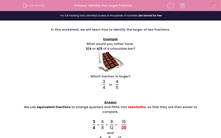# Identify the Larger Fraction

In this worksheet, students must identify the larger of the two given fractions. Equivalent fractions or common sense may be used.Key stage:  KS 2

Curriculum topic:   Maths and Numerical Reasoning

Curriculum subtopic:   Fractions

Difficulty level:#### Worksheet Overview

In this worksheet, we will learn how to identify the larger of two fractions.

Example

What would you rather have:

3/4 or 4/5 of a chocolate bar?Which fraction is larger?

 3 4
or
 4 5

We use equivalent fractions to change quarters and fifths into twentieths, so that they are then easier to compare.

 3 4
=
 6 8
=
 9 12
=
 15 20

and

 4 5
=
 8 10
=
 16 20

Now we can see:

 16 20
is larger than
 15 20

and so

 4 5
>
 3 4

Sometimes we can use 'common sense'.

Remember, for example, that 1/3 is bigger than 1/4.

### What is EdPlace?

We're your National Curriculum aligned online education content provider helping each child succeed in English, maths and science from year 1 to GCSE. With an EdPlace account you’ll be able to track and measure progress, helping each child achieve their best. We build confidence and attainment by personalising each child’s learning at a level that suits them.

Get started